# Pathfinding꞉ BFS Algorithm

The Idea of pathfinding is simpler than it might seem.

Here's the problem:
you have a starting point (e.g. a player)
you know what you want to get to (e.g. a key)
you don't know where it is
you want the shortest path between the two
there may be obstacles that must be avoided

the solution is to use an ALGORITHM (a set of steps) to find the path.
the algorithm we'll be looking at is the BREADTH FIRST SEARCH (BFS) It looks like this: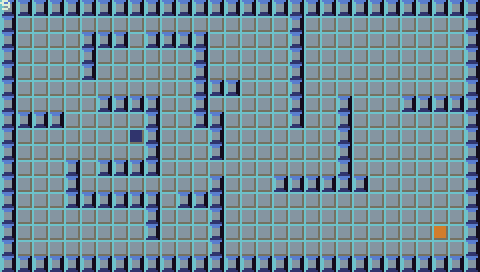You can play the demo here

As you can see it "flood fills" the level, then returns a path.
The blue tile is the starting point
the orange tile is the goal
the red tiles are the "closed" tiles (those that have already been checked)
the green tiles are the "open" tiles (those that we're currently checking)
The green tiles show the "frontier" of the algorithm. (the edge of the searched area)

NOTE if you don't care how it works, and just want to use it in your own project...
then skip down to the IMPORTING AND USING BFS() section

Here's the algorithm:

1. label the starting point as the "current" tile
2. label the current tile as "closed"
3. label the unset tiles around "current" as "open"
(Unset means we ignore "open" tiles, "closed" tiles, and walls)
4. set their "parent" to current
5. while the open list isn't empty and the goal hasn't been found do:
a. cycle through the "open" tiles in the order they were logged
b. label the current "open" tile as "current" (starting point is no longer the current tile)
c. label the current tile as "closed"
d. label the tiles around "current" as open
e. set their "parent" to current
f. check if the current tile is the goal
6. while the path_end isn't at the starting point do:
a.path_end is goals "parent" tile
b.log path_end into path c.path_end is path_end's own parent
d.check if path_end is at the starting point

Don't worry if you don't follow this exactly...we're working towards it.
Here's some visual aids:
START AT THE STARTING POINT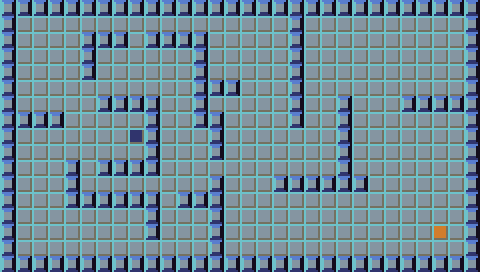LABEL THE SURROUNDING TILES TO OPEN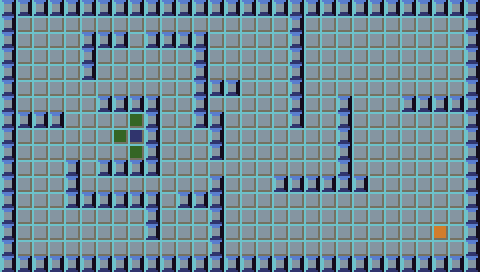THE TILES ARE NOW CLOSED AND ARROWS DRAWN TO SHOW PARENT ASSIGNMENT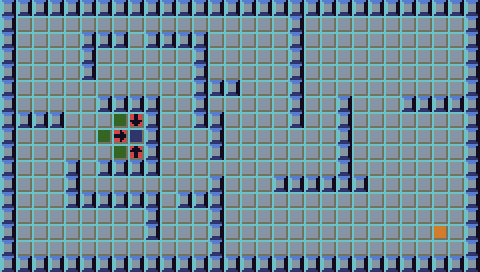SEVERAL CYCLES RAN NOW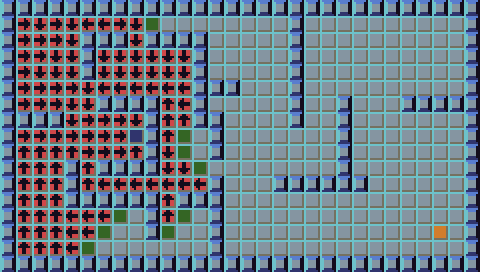Notice how the parent assignment works to build a path:
pick anywhere in the red area...
you will always arrive at the start!

Here's the algorithm running in slow motion: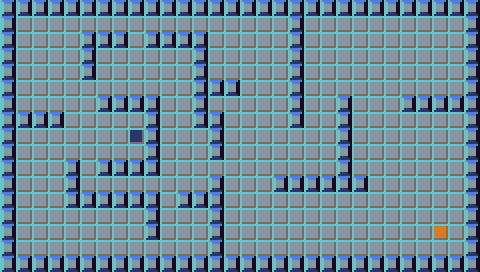## BFS in Lua

Now let's look at how we do this in TIC-80!

We'll create tables to keep track of "open" and "closed" tiles

``` local open  ={start}
local	closed={}```

BFS will be a self contained function that requires no external functions.
This means the few logic functions we need we will define within the main BFS() function.
This makes BFS() more portable.

```function bfs(a,b)
--we will refer to the (x,y) coors by
--number index so start is start.x
local start={a.x,a.y}
local goal ={b.x,b.y}

--a function to check if an item is
function is_in(item,list)
for i,ele in ipairs(list) do
if ele==item and ele==item then
return true
end
end
end

--a function to get the four adjacents
--to "cell" and log the valid ones
local up   ={cell  ,cell-1}
local down ={cell  ,cell+1}
local left ={cell-1,cell  }
local right={cell+1,cell  }

mget(v,v)<WALL then   --the adjacent isn't a wall

--build the object for logging
local z={v,v,cell}
--log it into open
table.insert(open,#open+1,z)
end
end
end```

Notice our tiles are logged with three attributes:

`z={x_coor, y_coor, parent_tile}  `

this way we can call them like so:

```z -- x coordinate
z -- y coordinate
z -- parent tile```

Now comes the BFS flood fill loop:

``` ::search::
--search as long as there's places to search
if #open>0 then

--since we will log the open tiles at the end of the "open" table...
--we only need to pull the tile from the first slot

--get the first cell out of open
current=open
table.remove(open,1)

--check if it's the goal
if current==goal and
current==goal then

--it it is goal then log the current tile as the end of the path
path_end=current
--also start the pathfinding code
mode="path"
end

--log its valid neighbors into open
--log current into closed
table.insert(closed,#closed+1,current)
end

--if we haven't found the goal then goto the start
--of the search loop
if mode=="search" then goto search end```

The last step is to run the pathbuilding code

``` --pathbuilding
::path_find::

--log the path_end into the start of path
--this way you log the return path in reverse so
--it can be followed.
table.insert(path,1,path_end)

--check if it HASN'T reached the start
if path_end~=start then

--set the path_end to its own parent tile
path_end=path_end --the parent is the third element
--continue the pathfinding code
goto path_find
end

--after the goal has been found, and the path has been built...
--return the path table.
return path
end```

## IMPORTING AND USING BFS()

Here I will walk you through getting this function running in your own project.

COPY THE BFS() FUNCTION copy the following code into your project:

```----------------------------------------
------BFS PATHFINDING ALGORITHM---------
----------------------------------------
function bfs(a,b)
local start={a.x,a.y}
local goal ={b.x,b.y}

local open  ={start}
local	closed={}
local	path  ={}
local mode="search"

--a function to check if an item is
function is_in(item,list)
for i,ele in ipairs(list) do
if ele==item and ele==item then
return true
end
end
end

--a function to get the four adjacents
--to "cell" and log the valid ones
local up   ={cell  ,cell-1}
local down ={cell  ,cell+1}
local left ={cell-1,cell  }
local right={cell+1,cell  }

if not is_in(v,closed) and
not is_in(v,open  ) and
mget(v,v)<WALL then

--build the object for logging
local z={v,v,cell}
--log it into open
table.insert(open,#open+1,z)
end
end
end

::search::
--search as long as there's places to search
if #open>0 then
--get the first cell out of open
current=open
table.remove(open,1)

--check if it's the goal
if current==goal and
current==goal then
path_end=current
mode="path"
end

--log its valid neighbors into open
--log current into closed
table.insert(closed,#closed+1,current)
end

if mode=="search" then goto search end

--pathbuilding
::path_find::
table.insert(path,#path+1,path_end)
if path_end~=start then
path_end=path_end
goto path_find
end

return path
end
----------------------------------------
--------END OF BFS ALGORITHM------------
----------------------------------------```

Now you will need your objects to have their attributes like this:

```--player object
p={
x=x_coor,
y=y_coor}

enemy={
x=x_coor,
y=y_coor}```

they can have as many attributes as you want, but bfs() specifically looks for attributes labeled
any_obj.x and
any_obj.y

now call the function like this:

`path=bfs(start_obj, goal_obj)`

path will be assigned a table of the steps needed to take to get to the goal.
the exact method you use to follow the path will depend on you're particular game.
for this reason I'll leave that up to you.

*NOTE This is not a very efficient method for pathfinding, but is useful if you don't know the exact placement of the goal, (like in a generated level) or you want to check every possible path in a level (like for checking if every section of the level is accessible) We will go over a more efficient method in an upcoming tutorial on A...

feel free to use any portion of this code in your own projects, just give me a credit at the top of your file and we're all good!

if you have questions, or requests for future tutorials, you can email me at bearknucklesketching@gmail.com

##### Clone this wiki locally
You can’t perform that action at this time.